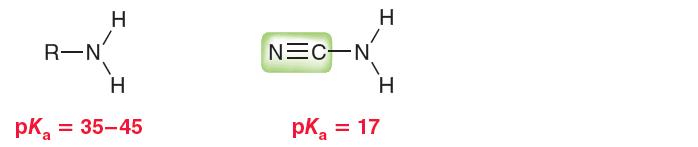Problem: Most common amines (RNH 2) exhibit pKa values between 35 and 45. R represents the rest of the compound (generally carbon and hydrogen atoms). However, when R is a cyano group, the pKa is found to be drastically lower: (b) Can you suggest a different replacement for R that would lead to an even stronger acid (pKa lower than 17)?

FREE Expert Solution
95% (329 ratings)
Problem Details

Most common amines (RNH 2) exhibit pKa values between 35 and 45. R represents the rest of the compound (generally carbon and hydrogen atoms). However, when R is a cyano group, the pKa is found to be drastically lower:

(b) Can you suggest a different replacement for R that would lead to an even stronger acid (pKa lower than 17)?What scientific concept do you need to know in order to solve this problem?

Our tutors have indicated that to solve this problem you will need to apply the Ranking Acidity concept. You can view video lessons to learn Ranking Acidity Or if you need more Ranking Acidity practice, you can also practice Ranking Acidity practice problems .

What is the difficulty of this problem?

Our tutors rated the difficulty of Most common amines (RNH 2) exhibit pKa values between 35 and... as high difficulty.

How long does this problem take to solve?

Our expert Organic tutor, Jonathan took less than a minute to solve this problem. You can follow their steps in the video explanation above.

What professor is this problem relevant for?

Based on our data, we think this problem is relevant for Professor Singh's class at QC CUNY .

What textbook is this problem found in?

Our data indicates that this problem or a close variation was asked in . You can also practice practice problems .